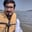Related Tags

python
pandas
columns
communitycreator

# How to change the order of columns of a dataframe in pandasArslan Bajwa

In Python, the pandas library has built-in functionalities to perform different tasks with only a few lines of code. One of these functionalities allows you to change the order of columns in dataframe.

## Method 1

In this method, we first initialize the pandas dataframe with a sample list format "abcde". Then, we use the list() function of Python to interchange the columns’ order, which will convert the given format into a specified format of list.

#importing pandas and numpy libraries
import pandas as pd
import numpy as np

#initializing pandas dataframe
df = pd.DataFrame(np.arange(30).reshape(-1, 5), columns=list('abcde'))

#changing order of column
df = df[list('cbdea')]
print(df)


## Method 2

In this method, we first initialise the pandas dataframe. Then, we use the tolist() function to convert the columns of the dataframe into a list. Afterwards, we find the index of the columns that we want to change in the dataframe. In the end, we simply swap the two columns.

#importing pandas and numpy libraries
import pandas as pd
import numpy as np

#initializing pandas dataframe
df = pd.DataFrame(np.arange(30).reshape(-1, 5), columns=list('abcde'))

#changing order of column
column1 = 'b'
column2 = 'd'
colnames = df.columns.tolist()
index1, index2 = colnames.index(column1), colnames.index(column2)
colnames[index2], colnames[index1] = colnames[index1], colnames[index2]
df = df[colnames]

print(df)


## Method 3

In this method, we first initialise the pandas dataframe. We can use the sorted() function of Python to sort the columns of the dataframe and change their order.

#importing pandas and numpy libraries
import pandas as pd
import numpy as np

#initializing pandas dataframe
df = pd.DataFrame(np.arange(30).reshape(-1, 5), columns=list('acdbe'))

#changing the order of column
df = df[sorted(df.columns)]
print(df)


## Method 4

In this method, we first initialise the pandas dataframe. Then, we apply the sort_index() function of Python to sort the columns of the dataframe. This function sorts in ascending/descending order and along a specified axis.

#importing pandas and numpy libraries
import pandas as pd
import numpy as np

#initializing pandas dataframe

#changing order of column
df.sort_index(axis=1, ascending=True, inplace=True)
print(df)


RELATED TAGS

python
pandas
columns
communitycreator

CONTRIBUTORArslan Bajwa
RELATED COURSES

View all Courses

Keep Exploring

Learn in-demand tech skills in half the time